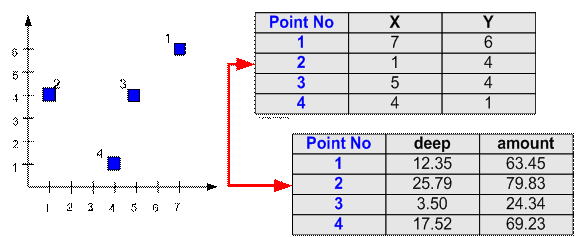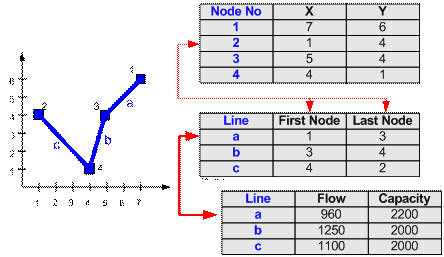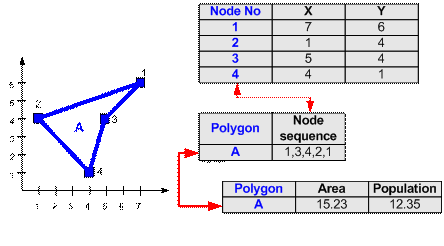The most common representation of a map is using vector data, that is consist of points, line and polygons.

### Point

Each point is stored by its location (X, Y) together with the table attribute of this point.

For example, 4 points below has their coordinate location in (X, Y) and each point has attributes of deep and amount of water contamination.### Line

Each line is stored by the sequence of first and last point together with the associated table attribute of this line. For example, three lines below (a, b and c) have their first and last node to distinguish their location and each line has attributes of flow and capacity of the sewerage pipe. Notice that each node has coordinate (X, Y) that is stored in another table.Because the first and end node coordinates of each line is known, the length of a line or poly-line (sequence of lines) can be easily computed.

In most GIS software nowadays, you can use Arc instead of line. The representation is actually the same with additional tangent and length of tangent of the two ends.

### Polygon

Polygon is represented by a closed sequence of lines. Unlike line or poly-line (sequence of line), polygon always closed. That is, the first point is equal to the last point. A polygon can be represented by a sequence of nodes where the last node is equal to the first node. For example, polygon A below has its first and last node in node number 1 to settle its location. Aside from location attributes, the polygon has associated attributes of area and bacterial population. Notice that each node has coordinate (X, Y) that is stored in another table.Using polygon, several geometric attributes such as area and perimeters can be derived easily.

### What things do we represent as Point, Line or Polygon?

Data representation is depending on

1. Map scale , and
2. Functions you want to perform in your later analysis.

What things represented as line (or poly-line) may be easy to guess: road, pipeline, water line, rivers, bus route and so on that have basic shape similar to line or combination of lines.

What things we represent as a point or polygon? In the city map scale around 1:25,000 or 1:10,000; you may represent buildings, post offices, bus stops, hospitals, police stations, wells, and so on as points. If you need more detail map, however, say in the scale of 1:1000, those infrastructure listed above may be better to be represented as polygons, rather than point.

Point is simpler to input and analyzed. Polygon need more points to input but you may get the area, perimeter and other geometric attributes computed by the GIS software rather than you input manually. If you are sure that you do not need these geometrical attributes in your later analysis, input your data as point rather than polygon.

Preferable reference for this tutorial is

Teknomo, Kardi (2015) Introduction to GIS. https:\\people.revoledu.com\kardi\ tutorial\GIS\